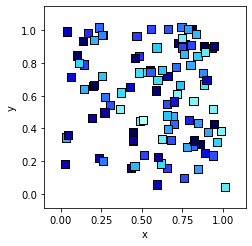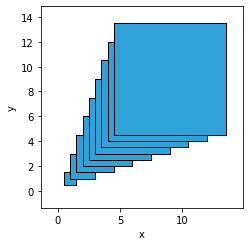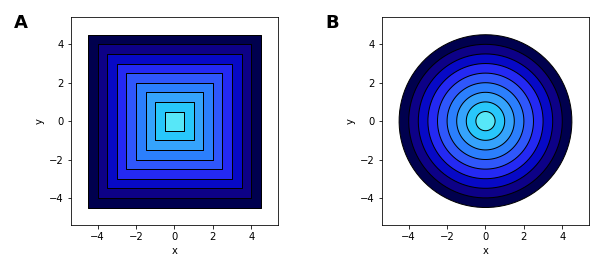# Polygons#

Title
Polygons Element
Dependencies
Matplotlib
Backends
Matplotlib
Bokeh
```import numpy as np
import holoviews as hv
hv.extension('matplotlib')
```A `Polygons` represents a contiguous filled area in a 2D space as a list of polygon geometries. Just like the `Contours` element additional scalar value dimensions maybe may be supplied, which can be used to color the `Polygons` with the defined `cmap`. Like other `Path` types it accepts a list of arrays, dataframes, a dictionary of columns (or any of the other literal formats including tuples of columns and lists of tuples), but also supports a special ‘holes’ key to represent empty interior regions. For a full description of the polygon geometry data model see the Geometry Data User Guide.

In order to efficiently represent the scalar values associated with each path the dictionary format is preferable since it can store the scalar values without expanding them into a whole column. Additionally it allows passing multiple columns as a single array by specifying the dimension names as a tuple.

In this example we will create a list of random polygons each with an associated `level` value. Polygons will default to using the first value dimension as the `color` but for clarity we will define the `color` explicitly:

```def rectangle(x=0, y=0, width=.05, height=.05):
return np.array([(x,y), (x+width, y), (x+width, y+height), (x, y+height)])

polys = hv.Polygons([{('x', 'y'): rectangle(x, y), 'level': z}
for x, y, z in np.random.rand(100, 3)], vdims='level')

polys.opts(color='level', linewidth=1)
````Polygons` is a very versatile element which may be used to draw custom annotations, choropleth maps (as can be seen in the texas_unemploment example) among many other examples. We can also use some of the other path based annotations to quickly generate polygons, including `Box`, `Bounds` and `Ellipse` elements. In the simple case we can simply pass a list of these elements:

```hv.Polygons([hv.Box(i, i, i) for i in range(10)])
```Alternatively we can use the `array` method to return the x/y-coordinates of the annotations and define additional z-values by declaring a dictionary:

```hv.Polygons([{('x', 'y'): hv.Box(0, 0, i).array(), 'z': i} for i in range(10)[::-1]], vdims='z') +\
hv.Polygons([{('x', 'y'): hv.Ellipse(0, 0, (i, i)).array(), 'z': i} for i in range(10)[::-1]], vdims='z')
```For full documentation and the available style and plot options, use `hv.help(hv.Polygons).`

This web page was generated from a Jupyter notebook and not all interactivity will work on this website. Right click to download and run locally for full Python-backed interactivity.